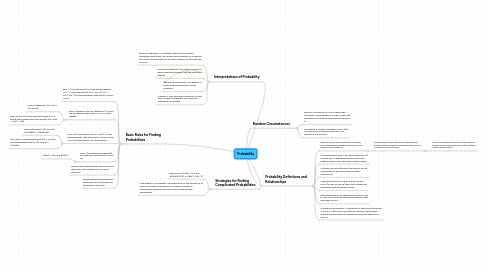Probability

Get Started. It's FreeProbability3. Probability Definitions and Relationships

3.1. Sample space: the collection of unique, non-overlapping possible outcomes of a random circumstance.

3.1.1. Simple Event: one outcome in the sample space. Simple event is a possible outcome of a random circumstance.

3.1.1.1. Event: a collection of one or more simple events in the sample space. Often written using capital letters.

4. Basic Rules for Finding Probabilities

4.2. Rule 2 (addition rule for "either/or"): to find the probability that either A or B or both happen.

4.2.1. Rule 2a (general): P(A or B) = P(A and B)

4.2.2. Rule 2b (for mutually exclusive events): if A and B are mutually exclusive events, P(A or B) = P(A) + P(B)

4.3. Rule 3 (multiplication rule for "and"): to find the probability that two events A and B both occur simultaneously or in a sequence.

4.3.1. Rule 3a (general): P(A and B) = P(A)P(B|A) = P(B)P(A|B)

4.3.2. Rule 3b (for independent events): if A and B are independent events, P(A and B) = P(A)P(B)

4.4. Rule 4 (conditional probability): an algebraic restatement of rule 3a

4.4.1. P(B|A) = P(A and B)/P(A)